# Moving Elements by SymmetryThis task shows you how to move existing Sketcher elements using a line, a construction line or an axis. In this particular case we will move a rectangle by symmetry.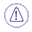The former functionality associated to this command is available thru the Mirror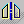command, which duplicates elements by symmetry.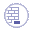1. Create a rectangle and an axis.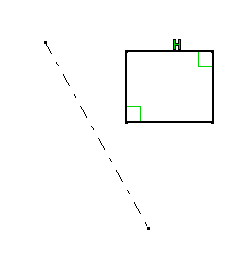2. Click Symmetry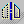from the Transformation sub-toolbar in the Operation toolbar.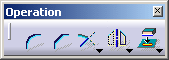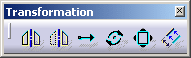3. Select the rectangle you have created and click on the axis.
The rectangle has been moved by symmetry according to the axis.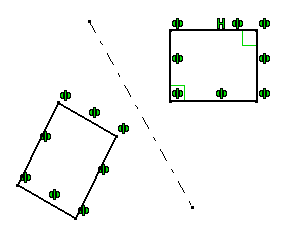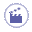## Two sides selection

1. Create an axis.

2. Create a rectangle on one side of the Axis and a circle on the other side.1. Click Symmetryfrom the Transformation sub-toolbar in the Operation toolbar.1. Select the rectangle and the circle by trapping.

2. Select the axis.
In order to be able to multi-select elements, the axis length must be quite important.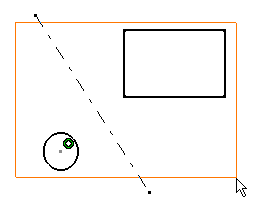The symmetry is created and the two elements have been taken into account.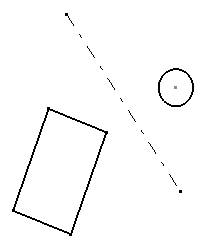## Applying constraints to symmetrical elements

1. Create a rectangle and an axis.

1. Select Constraint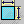from the Constraint toolbar.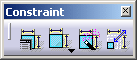1. Select one of the rectangle element and the axis.

2. Click to create the constraint.
The constraint and its value are displayed in the geometry area.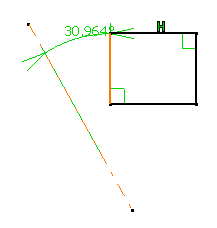1. Click Symmetryfrom the Transformation sub-toolbar in the Operation toolbar.2. Select the rectangle and then click on the axis.
The rectangle has been moved by symmetry according to the axis.
Note that as the constraint is applied on an axis, the constraint is kept after the symmetry.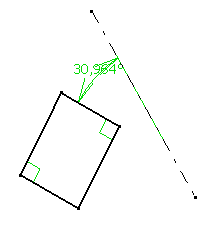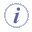• The constraint is also kept when it is applied to a fixed element.
• In the case of Use Edges, the element becomes isolated.Only internal constraints are kept after a symmetry operation.